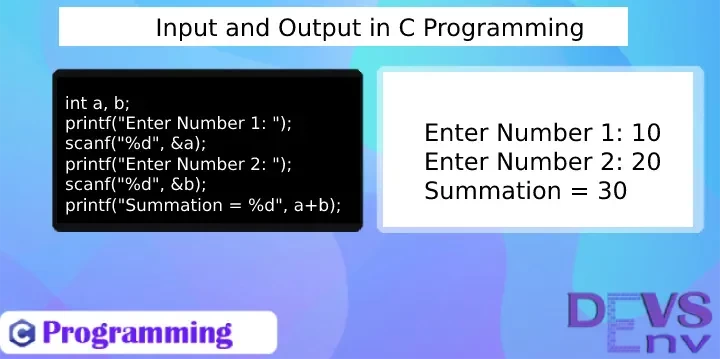Categories - C Programming Tags - C++ C   Maniruzzaman Akash   2 years ago   3286   4 minutes   0

# #3 Input and Output in C Programming

Output: We want to print/output anything in C Program.

We've already the basic concept of data types and also get some example of print there. Output means - something print in stdout. We want to print something in C program, so how to do that.

Function name: `printf()`

`printf()` is a library function of C, which sends a formatted output to stdout.

Basic printf() function - `int printf(const char *format, ...)`

Ok, Let's take some practical example of printing in C programming.

Problem 1: We want to print Hello World.

``printf("Hello World");``

So, here, we've just pass a string as argument and it'll send it to the stdout and it prints - Hello World.

Problem 2: We want to make calculation of two numbers and then print the summation.

``````int a = 10, b = 20;
printf("Summation = %d", a+b); // Output: Summation = 30``````

We've used `%d` as the output variable is integer. We've learned that in previous lesson.

Problem 3: We want to make division of two float numbers and then print the division.

``````float a = 45.5, b = 5.5;
printf("Division = %f", a/b); // Output: Division = 8.272727``````

We've used %f because, it is float type value. We've learned that in previous lesson.

Complete Example of summation and division with printing.

``````#include <stdio.h>

int main(){
int number1 = 10, number2 = 20, summation;
summation = number1 + number2;
printf("Summation = %d\n", summation); // Output: Summation = 30

float number3 = 45.5, number4 = 5.5, division;
division = number3 / number4;
printf("Division = %f\n", number3/number4); // Output: Division = 8.272727
}
``````

Look at the function now - `printf("Division = %f\n", number3/number4)`

We've used `%f\n`, here `\n` is for new line. And it's a common practice and standard to give new line after a print.

Input: We want to take input from C Program.

Function Name: `scanf()`

`scanf()` is a library function in C, which reads input from stdin. Actually it accepts two types of things -

1. Format to be taken
2. Value to be taken

Basic scanf() - `int scanf(const char *format, ...)`

Practical Example of scanf() :

Problem 1: We want to give a number input and we'll print that number.

``````#include <stdio.h>

int main(){
int number;

scanf("%d", &number);

printf("Number is: %d", number);
return 0;
}
``````

Line By Line Code Description of this input:

1. First, we print - Please Enter Number:
2. Then we use - `scanf("%d", &number);`
1. We take a number from input using this.
2. We've used %d, cause it's a decimal/integer value
3. Then we do - `printf("Number is: %d", number);`
1. We print a decimal number and
2. It'll just print our given number to the input.
4. Finish program - `return 0;`

Problem 2: We want to give two number input and we'll print the summation to the output.

``````#include <stdio.h>

int main(){
int number1, number2, summation;

scanf("%d", &number1);

scanf("%d", &number2);

summation = number1 + number2;

printf("Summation is: %d", summation);
return 0;
}
``````

In here we combined our `printf()` and `scanf()` function to do at a time input and output in C programming.

Problem 3: We want to give multiple number same time in input and we'll print the summation to the output.

``````#include <stdio.h>

int main(){
int number1, number2, summation;

printf("Please Enter Number 1 and 2: ");
scanf("%d %d", &number1, &number2);

summation = number1 + number2;

printf("Summation is: %d", summation);
return 0;
}
``````

So, look at this line, how to take multiple input in C programming -

``scanf("%d %d", &number1, &number2);``

So, this way, we can take any numbers if we want.

Problem 4: We want to give first name and last name to the input and print the full name.

``````#include <stdio.h>

int main(){
char firstName, lastName;

scanf("%s", firstName);

scanf("%s", lastName);

printf("Full Name: %s %s\n", firstName, lastName);
return 0;
}
``````

So, as it's string we've used %s.

And one more different it's from the previous example. Look in previous example we've used `&d`, but here only `s`.

So, we've got enough problem and examples of input and output of C programming. Hope now, it's easy to understand how input and output works in C programming.

Basic and popular format identifiers in C.

Data Type Format Specifier
`int` `%d`
`char` `%c`
`float` `%f`
`double` `%lf`
`short int` `%hd`
`unsigned int` `%u`
`long int` `%li`
`long long int` `%lli`
`unsigned long int` `%lu`
`unsigned long long int` `%llu`
`signed char` `%c`
`unsigned char` `%c`
`long double` `%Lf`

Comment after signing in, if anything missed about input and output of C programming.

Related Live Coding Examples for testing input/output:

Get All Programming Example in C Programming Category - Go Link

Tags: C Programming input output, how to take input in c, c input, c output, how to print in c, c programming print, c programming input, scanf() in c, printf() in c, scanf() function details, printf() function details, c programming examplem c examples.